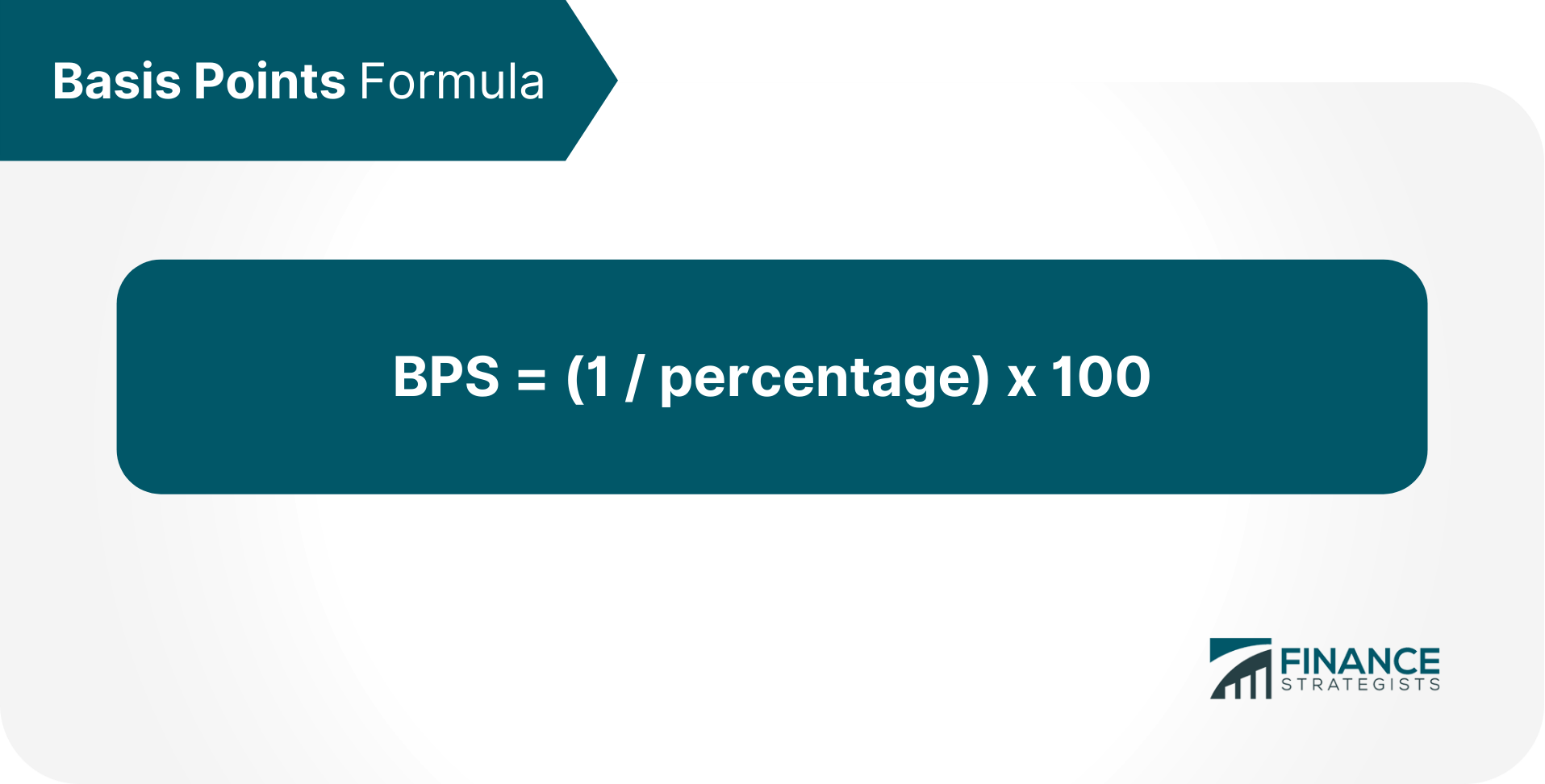# Basis Points (BPS)### Written byTrue Tamplin, BSc, CEPF® | Reviewed by Editorial Team

Updated on January 04, 2023

Basis Points (BPS) are a measure of change in a financial instrument.

They are usually used when quoting yield spreads, interest rates, such as the prime rate or LIBOR. Because interest rates are typically quoted as annual rates, basis points are also commonly referred to as bps or bp.

One basis point is equivalent to 1/100th of a percent (0.01%), or 0.0001 in decimal form. So, if an interest rate changes from 5% to 5.01%, that rate has increased by 1 basis point.

## The Importance of Basis Points

The importance of basis points is in how they provide a standardized way to compare different rates of return.

Since interest rates are typically measured in annual percentages, businesses can use basis points to make easier comparisons between short-term and long-term investments, for example. With the ability to directly compare returns across different time periods, businesses can make more informed investment decisions.

In addition, because basis points are also a percentage of the rate being measured, they can be helpful in indicating just how much a change in a rate will affect the overall return on investment.

For example, a 1 basis point change in a 5% interest rate will result in a 0.05% change in the return on investment.

## Basis Points Formula

The basis points formula is:In other words, to calculate basis points, divide 1 by the interest rate, and then multiply by 100.

Consider the example of an interest rate increase from 5% to 5.01%.

The basis point formula would be: BPS = (1 / 0.05) x 100 = 200 BPS So, this increase of 1 basis point is equal to an increase of 200 basis points.

## Why Use Basis Points Versus Percentages?

While basis points can be confusing to some, they are the standard unit of measure for most currencies around the world.

This consistency allows businesses to accurately compare returns across different time periods and different currencies.

## How to Use Basis Points in Practice

Now that you understand what basis points are and how to calculate them, let's look at how they can be used in practice.

One common use of basis points is in the calculation of yield spreads. A yield spread is a difference between two interest rates, typically the rate offered on a savings account or certificate of deposit (CD) and the rate offered on a loan, such as a mortgage.

The lower of the two interest rates is used as the denominator in the basis points calculation. For example, if the interest rate on a savings account is 2% and the interest rate on a loan is 8%, the yield spread is 6%.

To calculate the basis points for this yield spread, use the following formula: BPS = (1 / 0.06) x 100 = 1667 BPS So, this 6% yield spread is equivalent to an increase of 1,667 basis points.

Basis points can also be used when calculating risk premiums for different types of investments.

For example, the Capital Asset Pricing Model (CAPM) uses basis points in its formula to calculate the required rate of return for a given investment.

### Impact on Returns

Basis points are also useful for understanding how a change in a rate will affect the overall return on investment.

For example, if the interest rate on a five-year bond increases from 5% to 6%, that's an increase of 1 percentage point or 100 basis points. If you have a \$1,000 investment in this bond, your investment would now be worth \$1,100.

With the increase in your rate of return, you could compare the new value of your investment to other investments with similar risk profiles and maturity dates.

Basis points are often used by mortgage lenders when quoting interest rates on loans.

For instance, if someone is purchasing a million-dollar home and the lender quotes a rate of 4.5%, that's equivalent to an annual interest rate of 0.045 or 4.5 basis points (bps) on a daily basis.

In other words, for every \$1,000 you borrow, you will pay an extra \$4.50 in interest each year.

## The Bottom Line

Basis points are a measure of change in an interest rate or yield.

They can be used to compare different investments, calculate yield spreads, and understand the impact of a change in interest rates on returns. Understanding what they are and how they're used can give you a better understanding of the financial markets and help you make more informed investment decisions.

## Basis Points (BPS) FAQs

### What are the basis points?

Basis points are a measure of change in an interest rate or yield. This means that when an interest rate or yield changes, the number of basis points it has changed by is also affected.

### How do I calculate basis points?

To calculate basis points, divide 1 by the interest rate, and then multiply by 100. The formula looks like this: Basis Points = 1 / Interest Rate * 100.

### Are basis points good for comparing different types of investments?

Yes, basis points are useful for comparing different types of investments. This is because basis points can be calculated and used to compare any type of interest rate or yield.

### Why use basis points versus percentages?

Basis points are used instead of percentages because they provide a more precise measure of change. This is especially helpful when comparing investments with different maturities.

### How can I use basis points in practice?

There are a few ways that you can use basis points in practice. One way is to compare the yield on two different investments that have similar risk profiles or maturities. Another way to use basis points is to calculate the impact of a change in an interest rate on your overall return. In this case, you can use the amount as a basis for comparison with other similar investments.